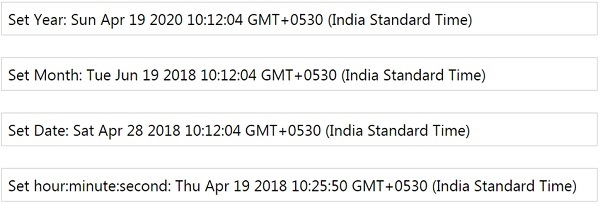# MomentJS - Set

This method will set the unit with the value provided. The unit is the first param and the value as the second. It can also take the unit, value pair in object form.

## Syntax

```moment().set(String, Int);
moment().set(Object(String, Int));
```

## Example

```var m = moment().set('year', 2020);
var d = moment().set('month', 5);
var a = moment().set('date', 28);
var k = moment().set({hour:10, minute:25, second:50});
```

## Outputmomentjs_getter_setter.htm